# Resources tagged with: Multiplication & division

Filter by: Content type:
Age range:
Challenge level:

### There are 147 results

Broad Topics > Calculations and Numerical Methods > Multiplication & division### Divide it Out

##### Age 7 to 11 Challenge Level:

What is the lowest number which always leaves a remainder of 1 when divided by each of the numbers from 2 to 10?### Ducking and Dividing

##### Age 7 to 11 Challenge Level:

Your vessel, the Starship Diophantus, has become damaged in deep space. Can you use your knowledge of times tables and some lightning reflexes to survive?### Tom's Number

##### Age 7 to 11 Challenge Level:

Work out Tom's number from the answers he gives his friend. He will only answer 'yes' or 'no'.### Oh! Hidden Inside?

##### Age 11 to 14 Challenge Level:

Find the number which has 8 divisors, such that the product of the divisors is 331776.### Journeys on the Gattegno Tens Chart

##### Age 5 to 11

Alf describes how the Gattegno chart helped a class of 7-9 year olds gain an awareness of place value and of the inverse relationship between multiplication and division.### Powerful Factorial

##### Age 11 to 14 Challenge Level:

6! = 6 x 5 x 4 x 3 x 2 x 1. The highest power of 2 that divides exactly into 6! is 4 since (6!) / (2^4 ) = 45. What is the highest power of two that divides exactly into 100!?### Eminit

##### Age 11 to 14 Challenge Level:

The number 8888...88M9999...99 is divisible by 7 and it starts with the digit 8 repeated 50 times and ends with the digit 9 repeated 50 times. What is the value of the digit M?### A First Product Sudoku

##### Age 11 to 14 Challenge Level:

Given the products of adjacent cells, can you complete this Sudoku?### Factoring Factorials

##### Age 11 to 14 Challenge Level:

Find the highest power of 11 that will divide into 1000! exactly.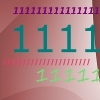### Ones Only

##### Age 11 to 14 Challenge Level:

Find the smallest whole number which, when mutiplied by 7, gives a product consisting entirely of ones.### Multiples Sudoku

##### Age 11 to 14 Challenge Level:

Each clue in this Sudoku is the product of the two numbers in adjacent cells.### Being Resilient - Primary Number

##### Age 5 to 11 Challenge Level:

Number problems at primary level that may require resilience.### What Two ...?

##### Age 7 to 11 Short Challenge Level:

56 406 is the product of two consecutive numbers. What are these two numbers?### What Is Ziffle?

##### Age 7 to 11 Challenge Level:

Can you work out what a ziffle is on the planet Zargon?### Missing Multipliers

##### Age 7 to 14 Challenge Level:

What is the smallest number of answers you need to reveal in order to work out the missing headers?### Remainders

##### Age 7 to 14 Challenge Level:

I'm thinking of a number. My number is both a multiple of 5 and a multiple of 6. What could my number be?### Repeaters

##### Age 11 to 14 Challenge Level:

Choose any 3 digits and make a 6 digit number by repeating the 3 digits in the same order (e.g. 594594). Explain why whatever digits you choose the number will always be divisible by 7, 11 and 13.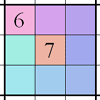### Integrated Product Sudoku

##### Age 11 to 16 Challenge Level:

This Sudoku puzzle can be solved with the help of small clue-numbers on the border lines between pairs of neighbouring squares of the grid.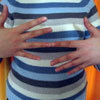### What Is the Question?

##### Age 11 to 16 Challenge Level:

These pictures and answers leave the viewer with the problem "What is the Question". Can you give the question and how the answer follows?##### Age 11 to 14 Challenge Level:

Visitors to Earth from the distant planet of Zub-Zorna were amazed when they found out that when the digits in this multiplication were reversed, the answer was the same! Find a way to explain. . . .### Difficulties with Division

##### Age 5 to 11

This article for teachers looks at how teachers can use problems from the NRICH site to help them teach division.### Looking at Lego

##### Age 7 to 11 Challenge Level:

This task offers an opportunity to explore all sorts of number relationships, but particularly multiplication.##### Age 11 to 14 Challenge Level:

If you take a three by three square on a 1-10 addition square and multiply the diagonally opposite numbers together, what is the difference between these products. Why?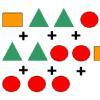### Super Shapes

##### Age 7 to 11 Short Challenge Level:

The value of the circle changes in each of the following problems. Can you discover its value in each problem?### Number Tracks

##### Age 7 to 11 Challenge Level:

Ben’s class were cutting up number tracks. First they cut them into twos and added up the numbers on each piece. What patterns could they see?##### Age 11 to 14 Challenge Level:

Make a set of numbers that use all the digits from 1 to 9, once and once only. Add them up. The result is divisible by 9. Add each of the digits in the new number. What is their sum? Now try some. . . .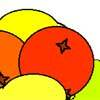### Oranges and Lemons

##### Age 7 to 11 Challenge Level:

On the table there is a pile of oranges and lemons that weighs exactly one kilogram. Using the information, can you work out how many lemons there are?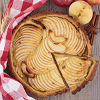### Pies

##### Age 7 to 11 Challenge Level:

Grandma found her pie balanced on the scale with two weights and a quarter of a pie. So how heavy was each pie?### Odds and Threes

##### Age 7 to 11 Challenge Level:

A game for 2 people using a pack of cards Turn over 2 cards and try to make an odd number or a multiple of 3.### The Remainders Game

##### Age 7 to 14 Challenge Level:

Play this game and see if you can figure out the computer's chosen number.##### Age 11 to 14 Challenge Level:

Powers of numbers behave in surprising ways. Take a look at some of these and try to explain why they are true.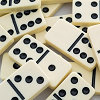### Amy's Dominoes

##### Age 7 to 11 Challenge Level:

Amy has a box containing domino pieces but she does not think it is a complete set. Which of her domino pieces are missing?### A Mixed-up Clock

##### Age 7 to 11 Challenge Level:

There is a clock-face where the numbers have become all mixed up. Can you find out where all the numbers have got to from these ten statements?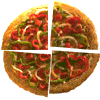### Fair Feast

##### Age 5 to 11 Challenge Level:

Here is a picnic that Petros and Michael are going to share equally. Can you tell us what each of them will have?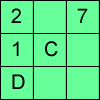### Alphabetti Sudoku

##### Age 11 to 16 Challenge Level:

This Sudoku requires you to do some working backwards before working forwards.### Double Digit

##### Age 11 to 14 Challenge Level: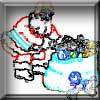### Clever Santa

##### Age 7 to 11 Challenge Level:

All the girls would like a puzzle each for Christmas and all the boys would like a book each. Solve the riddle to find out how many puzzles and books Santa left.### Book Codes

##### Age 7 to 11 Challenge Level:

Look on the back of any modern book and you will find an ISBN code. Take this code and calculate this sum in the way shown. Can you see what the answers always have in common?### Escape from the Castle

##### Age 7 to 11 Challenge Level:

Skippy and Anna are locked in a room in a large castle. The key to that room, and all the other rooms, is a number. The numbers are locked away in a problem. Can you help them to get out?### Times Right

##### Age 11 to 16 Challenge Level:

Using the digits 1, 2, 3, 4, 5, 6, 7 and 8, mulitply a two two digit numbers are multiplied to give a four digit number, so that the expression is correct. How many different solutions can you find?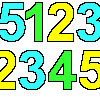### 1, 2, 3, 4, 5

##### Age 7 to 11 Challenge Level:

Using the numbers 1, 2, 3, 4 and 5 once and only once, and the operations x and ÷ once and only once, what is the smallest whole number you can make?### Largest Product

##### Age 11 to 14 Challenge Level:

Which set of numbers that add to 10 have the largest product?### A Conversation Piece

##### Age 7 to 11 Challenge Level:

Take the number 6 469 693 230 and divide it by the first ten prime numbers and you'll find the most beautiful, most magic of all numbers. What is it?### The Genie in the Jar

##### Age 11 to 14 Challenge Level:

This jar used to hold perfumed oil. It contained enough oil to fill granid silver bottles. Each bottle held enough to fill ozvik golden goblets and each goblet held enough to fill vaswik crystal. . . .### Surprising Split

##### Age 7 to 11 Challenge Level:

Does this 'trick' for calculating multiples of 11 always work? Why or why not?### Numbers Numbers Everywhere!

##### Age 5 to 11

Bernard Bagnall recommends some primary school problems which use numbers from the environment around us, from clocks to house numbers.### Why 8?

##### Age 11 to 14 Challenge Level:

Choose any four consecutive even numbers. Multiply the two middle numbers together. Multiply the first and last numbers. Now subtract your second answer from the first. Try it with your own. . . .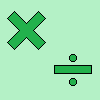### More Playing with Number Upper Primary

##### Age 7 to 11

Related resources supporting pupils' understanding of multiplication and division through playing with numbers.### Legs Eleven

##### Age 11 to 14 Challenge Level:

Take any four digit number. Move the first digit to the end and move the rest along. Now add your two numbers. Did you get a multiple of 11?### Like Powers

##### Age 11 to 14 Challenge Level:

Investigate $1^n + 19^n + 20^n + 51^n + 57^n + 80^n + 82^n$ and $2^n + 12^n + 31^n + 40^n + 69^n + 71^n + 85^n$ for different values of n.